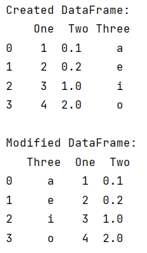# Set Order of Columns in Pandas DataFrame

Given a Pandas DataFrame, we have to set order of columns. By Pranit Sharma Last updated : September 23, 2023

Pandas is a special tool that allows us to perform complex manipulations of data effectively and efficiently. Inside pandas, we mainly deal with a dataset in the form of DataFrame. DataFrames are 2-dimensional data structures in pandas. DataFrames consist of rows, columns, and the data.

## Problem statement

Given a Pandas DataFrame, we have to set order of columns.

## Setting the Order of Columns in Pandas DataFrame

If you have a DataFrame with three columns named 'One', 'Two', and 'Three', in the same sequence and if you want to change the sequence of the columns, you need to pass the columns inside in a list in a sequence that you want to be.

Note

To work with pandas, we need to import pandas package first, below is the syntax:

```import pandas as pd
```

Let us understand with the help of an example,

## Python Code to Set Order of Columns in Pandas DataFrame

```# Importing pandas package
import pandas as pd

# Creating a dictionary
d = {
'One':[1,2,3,4],
'Two':[0.1,0.2,1,2],
'Three':['a','e','i','o']
}

# Creating dataframe
df = pd.DataFrame(d)

# Display DataFrame
print("Created DataFrame:\n",df,"\n")

# Reordering the columns
list = df[['Three', 'One', 'Two']]

# Display modified DataFrame
print("Modified DataFrame:\n",list)
```

### Output

The output of the above program is: